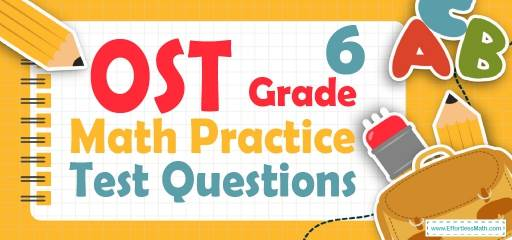# 6th Grade OST Math Practice Test QuestionsSometimes test takers think that they are 100% ready to answer and take the 6th Grade OST Math test by reading a 6th Grade math textbook. This idea is not entirely true! Participating in practice tests and solving sample questions is a necessity for a test taker and it can confront a test taker with what he or she will actually face in the test session. So here, our experts provide 10 6th Grade OST Math test problems along with a step-by-step guide to solving them that covers the most important math concepts in the 6th Grade OST Math test.

The Absolute Best Book to Ace the OST Math Test

## 10 Sample 6th Grade OST Math Practice Questions

1- What is the missing prime factor of the number 420?
$$420=2^2×3^1×…$$

A. $$2^2×3^1×5^1×7^1$$

B. $$2^2×3^1×7^1×9^1$$

C. $$1^2×2^3×2^1×3^1$$

D. $$3^2×5^1×7^1×9^1$$

2- If the area of the following trapezoid is equal to $$A$$, which equation represents $$x$$?

A. $$x = \frac{13}{A}$$

B. $$x = \frac{A}{13}$$

C. $$x=A+13$$

D. $$x=A-13$$

3- By what factor did the number below change from the first to the fourth number?
$$8, 104, 1352, 17576$$

A. 13

B. 96

C. 1456

D. 17568

4- 170 is equal to …

A. $$-20-(3×10)+(6×40)$$

B. $$((\frac{15}{8})×72 )+ (\frac{125}{5})$$

C. $$((\frac{30}{4} + \frac{15}{2})×8) – \frac{11}{2} + \frac{222}{4}$$

D. $$\frac{481}{6} + \frac{121}{3}+50$$

5- The distance between the two cities is 3,768 feet. What is the distance between the two cities in yards?

A. 1,256 yd

B. 11,304 yd

C. 45,216 yd

D. 3,768 yd

6- Mr. Jones saves $3,400 out of his monthly family income of$74,800. What fractional part of his income does Mr. Jones save?

A. $$\frac{1}{22}$$

B. $$\frac{1}{11}$$

C. $$\frac{3}{25}$$

D. $$\frac{2}{15}$$

7- What is the lowest common multiple of 12 and 20?

A. 60

B. 40

C. 20

D. 12

8- Based on the table below, which expression represents any value of f in terms of its corresponding value of $$x$$?

A. $$f=2x-\frac{3}{10}$$

B. $$f=x+\frac{3}{10}$$

C. $$f=2x+2 \frac{2}{5}$$

D. $$2x+\frac{3}{10}$$

9- 96 kg $$=$$… ?

A. 96 mg

B. 9,600 mg

C. 960,000 mg

D. 96,000,000 mg

10- Calculate the approximate area of the following circle? (the diameter is 25)

A. 78

B. 491

C. 157

D. 1963

## Best 6th Grade OST Math Prep Resource for 2022

1- A
$$420=2^2×3^1×5^1×7^1$$

2- B
The area of the trapezoid is: area= $$\frac{(base 1+base 2)}{2})×height= ((\frac{10 + 16}{2})x = A$$
$$→13x = A→x = \frac{A}{13}$$

3- A
$$\frac{104}{8}=13, \frac{1352}{104}=13, \frac{17576}{1352}=13$$
Therefore, the factor is 13

4- C
Simplify each option provided.
$$A. -20-(3×10)+(6×40)=-20-30+240=190$$
$$B. (\frac{15}{8})×72 + (\frac{125}{5}) =135+25=160$$
$$C. ((\frac{30}{4} + \frac{15}{2})×8) – \frac{11}{2} + \frac{222}{4} = ((\frac{30 + 30}{4})×8)- \frac{11}{2}+ \frac{111}{2}=(\frac{60}{4})×8) + \frac{100}{2}= 120 + 50 = 170$$this is the answer
$$D. \frac{481}{6} + \frac{121}{3}+50= \frac{481+242}{6}+50=120.5+50=170.5$$

5- A
1 yard $$=$$3 feet
Therefore, $$3,768 ft × \frac{1 \space yd }{3 \space ft}=1,256 \space yd$$

6- A
3,400 out of 74,800 equals to $$\frac{3,400}{74,800}=\frac{17}{374}=\frac{1}{22}$$

7- A
Prime factorizing of $$20=2×2×5$$
Prime factorizing of $$12=2×2×3$$
LCM$$=2×2×3×5=60$$

8- C
Plug in the value of $$x$$ into the function f. First, plug in 3.1 for $$x$$.
$$A. f=2x-\frac{3}{10}=2(3.1)-\frac{3}{10}=5.9≠8.6$$
$$B. f=x+\frac{3}{10}=3.1+\frac{3}{10}=3.4≠10.8$$
$$C. f=2x+2 \frac{2}{5}=2(3.1)+2 \frac{2}{5}=6.2+2.4=8.6$$
This is correct!
Plug in other values of $$x. x=4.2$$
$$f=2x+2\frac{2}{5} =2(4.2)+2.4=10.8$$
This one is also correct.
$$x=5.9$$
$$f=2x+2 \frac{2}{5}=2(5.9)+2.4=14.2$$
This one works too!
$$D. 2x+\frac{3}{10}=2(3.1)+\frac{3}{10}=6.5≠8.6$$

9- D
1 kg$$=$$ 1000 g and 1 g $$=$$ 1000 mg
96 kg$$=$$ 96 $$×$$ 1000 g $$=$$96 $$×$$ 1000 $$×$$ 1000 $$=$$96,000,000 mg

10- B
The diameter of a circle is twice the radius. Radius of the circle is $$\frac{25}{2}$$.
Area of a circle = $$πr^2=π(\frac{25}{2})^2=156.25π=156.25×3.14=490.625≅491$$

Looking for the best resource to help you succeed on the OST Math Grade 6 Math test?

The Most Comprehensive Review for 6th-Grade Students

### What people say about "6th Grade OST Math Practice Test Questions - Effortless Math: We Help Students Learn to LOVE Mathematics"?

No one replied yet.

X
51% OFF

Limited time only!

Save Over 51%

SAVE $15 It was$29.99 now it is \$14.99